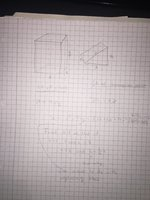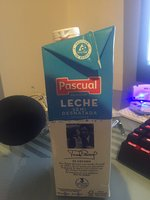# Optimizing the SA of an object

#### ImmmCanadian

##### New member
Hi I was wondering if anyone could help me in figuring out how to optimize the SA of an object, its for a project and im really confused. I am at only the early stages, but here is what I have attempted and the object I am optimizing.I wrote what I was confused about on the paper, but essentially I dont know how to get rid of y h and z and make the surface area in terms of x and finding the lowest SA, while maintaining a volume of 1000 cm^3. Thanks for any help

#### Dr.Peterson

##### Elite Member
I can't quite read everything you wrote (low contrast), but I hope you see that z can be expressed in terms of x and h, but x, y, and h are independent in determining the shape. The fixed volume allows you to eliminate one variable (say, h), expressing it in terms of the other two; but you still have the surface area as a function of two variables, x and y.

Are you studying multivariable calculus, or just calculus with a single independent variable? In the latter case, you would have to make some additional assumption in order to make this a problem you can solve; one way is to fix the angle of the top (that is, the ratio of h:r). Then you can express everything in terms of one variable. And you could then, if you wish, find the angle that optimizes the optimum (this is essentially equivalent to the multivariable method, using partial derivatives).

#### ImmmCanadian

##### New member
Thanks for the quick response. I am currently studying calculus with a single independent variable, and I am not sure how to fix the angle at the top, sorry.

If what my friend was saying is true, that if I take z=7,x=7,h=1, that i could express h=(1/7)x, then I believe it would be possible to make it into a singular variable. However I am not sure if he is correct.

Thanks again for any help/suggestions

#### Dr.Peterson

##### Elite Member
Thanks for the quick response. I am currently studying calculus with a single independent variable, and I am not sure how to fix the angle at the top, sorry.
My suggestion there was to choose a ratio h:x and make that a constant. For example, in the picture it looks like h = x/4, approximately. So just use that in place of h.

If what my friend was saying is true, that if I take z=7,x=7,h=1, that i could express h=(1/7)x, then I believe it would be possible to make it into a singular variable. However I am not sure if he is correct.
That doesn't even make sense. Since z is the hypotenuse of a right triangle with legs x and h, z can't be equal to x! But the result looks similar to what I just suggested, taking h as a particular fraction of x. (Then use the Pythagorean Theorem to find z.)

Now everything except y is expressed in terms of x, and you can use the given volume to express y in terms of x. Then write the SA as a function of x, and minimize it.

#### ImmmCanadian

##### New member
Thanks for all the help, I was able to finally get a value for x (although it was a painful process).